# Differential modeling flow: Development

## Flow considerations:

Two major considerations when developing a modeling flow are consistency and flexibility. This is particular true when it comes to differential buffer modeling. As discussed in our previous post, a half/true differential buffer goes through most of the same steps as single ended buffer yet certain process must be elaborated (e.g. modeling of the 2d surface sweep) and order needs to be preserved (i.e. extract C_Diff, I_Diff before performing VT simulation). In this post, we will briefly discuss how these considerations are incorporated into design concepts and realized in our SPIBPro modeling flow.

## Design setup:

Conceptually, user does not need to know whether a buffer is true/half/pseudo differential before modeling. The IBIS cookbook V4 also states this and also use encrypted hspice as an example… as it’s black box and can’t be deciphered, a model developer needs to assume coupling exists between P and N (thus true differential). The computed differential current from both 2D sweeps of IV and C_Diff will reveal whether such (true differential) assumption is valid. The decision can then be made based on the value of current.. if it’s in uA or nA range, then coupling is insignificant and can be modeled as pseudo differential.

In reality, we may argue that one should start with pseudo-differential approach and switch to half/full differential only if the final validation suggest so. This is because modeling engineer usually can get insights about buffer to be modeled by talking to the circuit designer (usually in the same company, also no needs to reveal or know much of the design details during such inquiry). In addition, the DC sweep of extra dimension and modelings of the half/true differential flow are often overkill for many of the designs.

In terms of modeling setup, only input and output (for N pin) are needed in addition to common single-ended buffer. A model type selection of “differential” is sufficient to indicate the differential modeling flow rather than linear single-ended modeling.## Modeling flow overview:

The modeling flow is summarized in the picture below: the key change here is that flow is not linear anymore. The simulation and post-processing stages need to be gone through twice. When simulating for the first time, only IV/C_Die sweep are needed. The data is then post-process for the first time to extract the common-mode and differential-mode current. The latter will also be modeled as a separated component in this stage. This differential component is then inserted between P and N pins in the transient netlist and simulated in the second iteration of the simulation step. However, only VT data is needed this time. When that’s done, all IV, VT and C-Die data are now available and the flow can continue linearly just like that of single-ended buffer.## Modeling of the IV data:

While PU/PD is steady state DC sweep in theory, their simulation is performed in “pseudo DC” most of the time due to likely existence of clock signal, thus not possible for true DC sweep. In “pseudo-DC” simulation, voltage are swept very slowly. In addition, sweeping along X coordinate of different Y values are mutually independent. So they can be simulated in parallel (multi threaded or can be distributed). Simulation under certain biasing condition may have convergence issue, so a flow must be tolerant about missing sweep data on some grid points due to non-convergence.

In our SPIBPro example below, sweep of different Y bias points (e.g. voltage at output N) are separated in different .sp files and they can be simulated in parallel.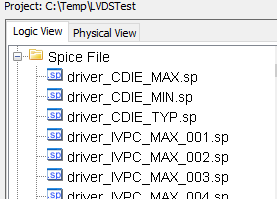The task of post-processing step in the first iteration is to extract the simulation data just swept, calculate common-mode current, shift the surface data vertically, summarized as a table output and also perform initial modeling. Such a table is important for user to validate the the result and also perform some what-if modeling using tool like Excel if neded.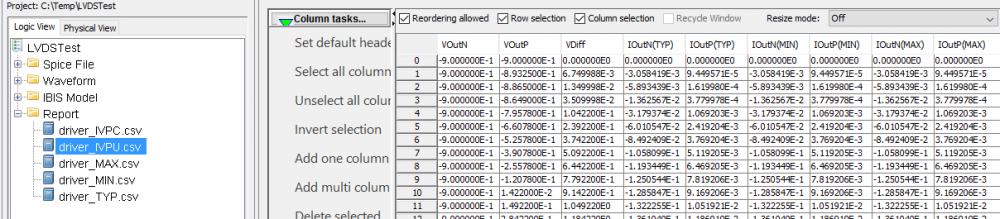• ## Surface modeling:

Depending on the symmetric differential mode data of shifted surface table (data on the surface alone the blue line), one may decide whether it’s pure resistive, linear or non-linear. The symmetric differential mode is orthogonal to the zeroed common mode curve (red straight line) and it represents the most likely differential operation (i.e. symmetric output of P and N)  For example, the surface plot below suggest a resistor will suffice for the content of the series element:With the csv table, one can quickly confirm whether the resistance is same across different voltage or not. If positive, then a linear resistor can be used. Otherwise, the non-linear resistance need to be modeled either with PWL spice element or as a series current (another IV table) in the series element. To be able to visualize the data with certain degrees of manipulation, a flow needs to have such 3D plotting capabilities. Otherwise, tool like matlab and familiarity of its syntax becomes necessary.If the sweeping data shows surface like above, then either a surface modeling is needed or a “series MOSFET” needs to be created  as a series element. For surface modeling, one can use “response surface modeling” like method to calculate fitting coefficients in minimizing the mean squared error sense. Other general modeling approach like neural network is certainly also possible.One should also check the residue of the prediction formula and maybe visualized as a scattered plot. SPIMPro’s general modeling and plotting functions are demonstrated below: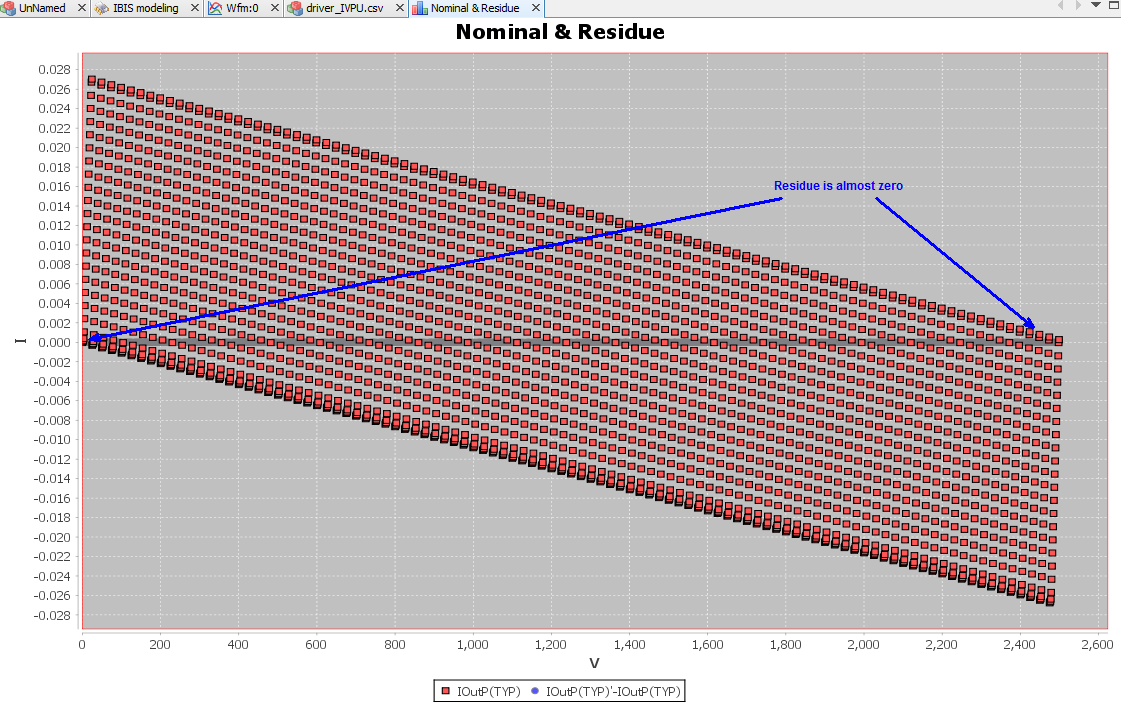A good fit should have very small residue, shown as grey line across 0.0 in the picture above (other red dots are nominal values from sweeping grids). With valid results, one will need to translate this “prediction formula” into spice netlist using E/F/G/H control elements.

In matlab, similar process can be done using “lsfit” function.

• Series element: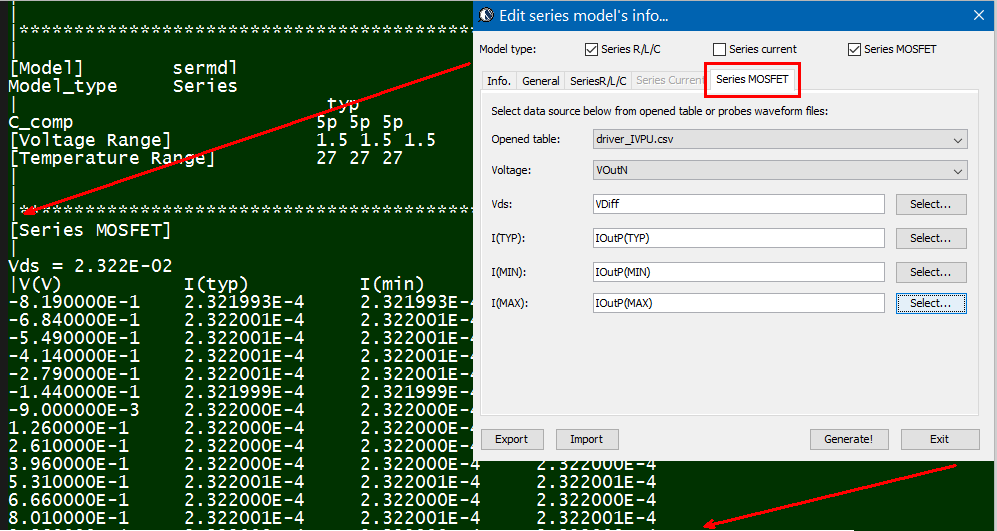The same table content is sufficient to construct a series element. The steps needed here is to translate data into a IBIS compatible format. Such process is trivial when the model is simply R/L/C. For series current and series MOSFET (can have up to 100 tables of different bias condition), the attention needed to perform such work manually is not economical and a tool/flow should be used instead.

## Modeling of the C-Diff:Similar process (tabulated and modeling) can be applied to calculated C_Diff. However, it’s much more limited in terms of series element’s syntax and E/F/G/H equation such that describing such surface (both frequency and voltage dependent) for C_Diff is not practical using either spice or series element. As a result, a trade-off needs to be made when picking values (or to average) from the surface and a single value is used when constructing model for C_Diff.

Verilog-A modeling:

In our submission to the IBIS summit later this year, we proposed another flow which is Verilog/VHDL based. One of the advantages of this implementation is that the raw, table-like data can be used directly using built-in \$table_model function. Its usage also enables polarity differentiation and elaborated description of C_Diff, which is very crucial to the transient data accuracy. The details about this will be published here once our paper is accepted.

Combined model:

In previous post, we mentioned that in addition to the “series element” for differential model, pure [External Model] of the differential model type may also be used. Using the proposed Verilog-A/VHDL for series element only model, these two methods can then work together, as shown below:A series model is still declared in the “[Series PIn Mapping]” section to be connected between buffer’s N and P outputs. Its definition, can optionally include an external model such as the one implemented with behavioral language. This “[external model]”  works on top of existing series model definitions and can provide extra info. such as frequency/voltage dependent C_Diff if the simulator supports. Optionally, the “top” series model can be simply a  shell (e.g. with high impedance) and all the info. regarding the differential current is encapsulated inside the added model. When comparing to external model attached under output/IO buffer, this external model can be significantly less complicated and also provide more tuning capabilities.

## Differential flow validation:

To validate a differential modeling flow,  one may construct a differential buffer by creating an artificial coupling (e.g. using some R/C elements) and then connect it between two known IBIS buffers. During the validation, the calculated common-mode data from DC sweep should reconstruct exactly the same PU/PD/PC/GC tables as those in the known IBIS buffer. Differential current will reveal the resitive element of the coupling portion. Calculated C_Diff should reveal the coupling capacitive element. Both of these steady state differential current and C_Diff can be validated using generated csv table or raw data. User should then find that with both captured accurately, the transient VT data calculated will also correlate to those of original IBIS buffer very well.

Paper and audio recording:

We presented this study at the 2016 Asian IBIS Summits. Readers may download the presentation from IBIS website or [HERE]. Audio recording at Tokyo is also available [HERE]

# Differential modeling flow: Revisit

In the past, we have blogged several articles regarding buffer modeling. The flow and focus there are mainly for single-ended. The considerations are that:

• Single ended (SE) buffer modeling is a good introduction to modeling flow in general without further complication;
• (Pseudo) differential buffer can actually be modeled as two single-ended buffers;
• Many (true/half) differential buffer can be modeled like pseudo differential buffer without significant loss of accuracy [LINK]
• Differential buffer modeling involve several steps which are a little more complicated.

During our workshops in Taiwan last month, several attendees asked about CML (current-mode logic) modeling. With the maturing of the concepts in SE modeling and the prevalence of SERDES interfaces such as USB, SATA, PCIe etc, we think it’s a good time to revisit the differential modeling flow in more details. We will also show how this design concepts are realized in our SPIBPro in next post.

Note that the contents of this and next posts are also written in conjunction with the paper submission to the IBIS summit later this year.

## Model descriptions:

[The info. here are summarized from the IBIS cookbook V4]

A differential buffer can be categorized into three different types:

• Pseudo differential: shown in the rightmost of the picture below. The “P” and “N” driving portion are mutually independent. There is no coupling between these two pins in the circuit.
• Half differential: shown in the middle. the pull-down portion of the “P” and “N” are coupled via a current source or shared load.
• True differential: shown in the leftmost. Both the pull-up and pull-down circuits are coupled via “current-mirror” and shared current source.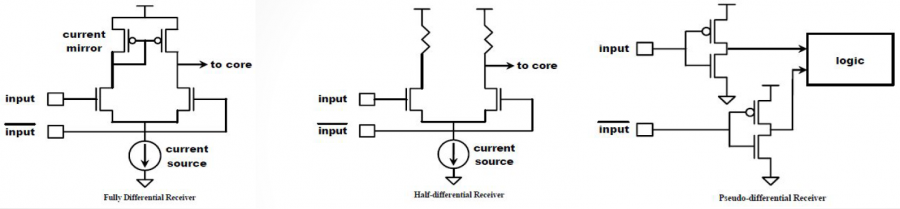For pseudo differential type buffer, the single ended buffer modeling flow can be applied directly. One only needs to generate these two “uncoupled” buffer separately (or use the same model with input flipped) and describe their differential behavior using the “diff pin” IBIS keyword: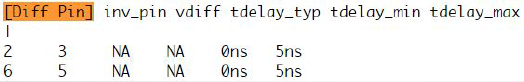Note that this “Diff Pin” keyword is also optional. So one may also simply instantiates two instance of the single ended buffer and use them together outside the IBIS file, such as in the spice netlist.

True/half differential buffer:

For a true/half differential buffers, the existence of “coupling” between P and N circuits must be described using existing IBIS syntax. There are two ways to do so: either using “series” or “external model” keywords:

• Series: There are two places series needs to be declared: the first one is “[series pin mapping]” in the model headers and the other one is “series model” of the “coupling” circuit in the “[model]” descriptions: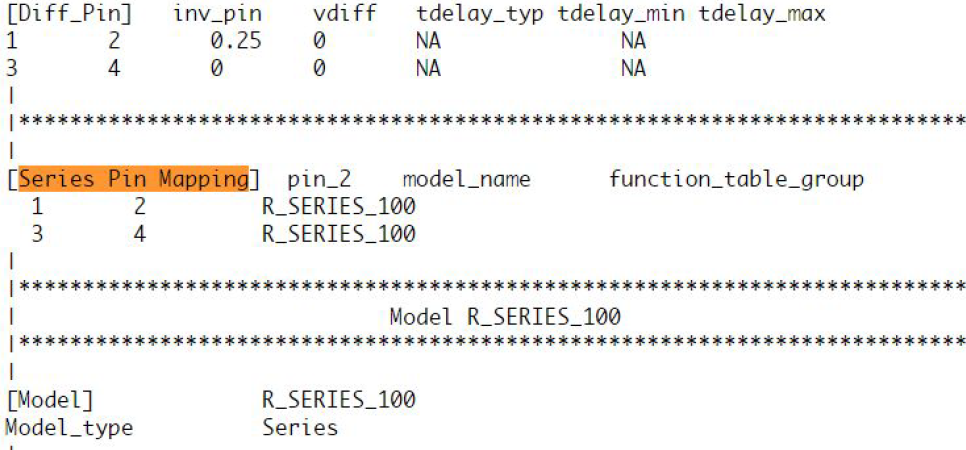Take the picture shown above as an example. Under the “Series Pin Mapping” keyword, a model named “R_SERIES_100” is declared to connect pin 1 to and 2. Another instance of such model also sit between pin 3 and 4. Pin 1~4 each has its own model defined in other part of the ibis file already. For example, pin 1, 2 may have an output buffer connected while pin 3, 4 have open-drain buffers. This R_SERIES_100 model must have type “Series” defined as part of the IBIS file. Since “Series” is one of predefined IBIS model type, its contents (keywords) are not free form and must be one or more of the following series elements: R, L, C, Series current and Series MOSFET which contains up to 100 I/V tables under different biasing voltages.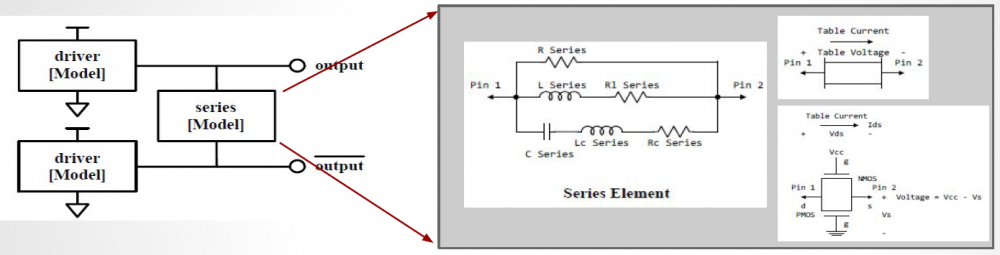• External Model:  In the IBIS spec, four different differential specific model types are also support.: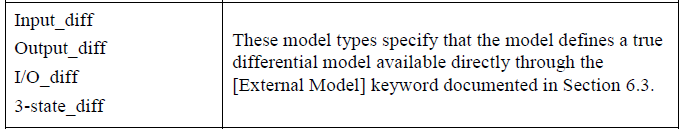These differential model types must be implemented with language such as Spice, VHDL-AMS, Verlog-AMS, IBIS Interconnect Spice Sub-circuits (IBIS ISS) and declared with the “External” model section. These languages provides much more flexibility in terms of modeling capabilities, yet they also diminish the portability of the generated IBIS model.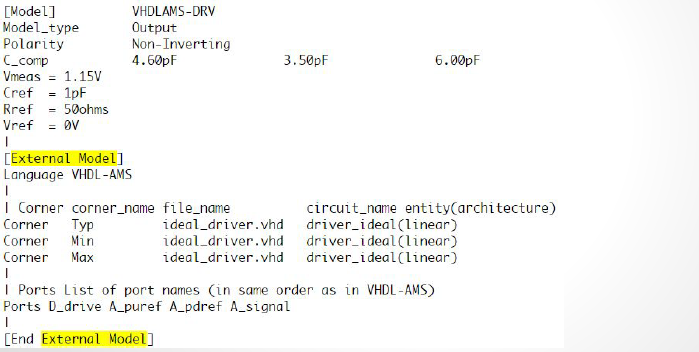In the example above, a separate file “ideal_driver.vhd” must be provided outside the IBIS file and an “entity” of name “driver_ideal” needs to be defined. The port connections is described using reserved keywords after the “Ports” statement. In the IBIS Spec, all possible ports are pre-defined and the declaring order here must match their definitions in the associated Verilog/VHDL etc file.

Modeling process:

Pure method 2 implementation, i.e. coding in language like Verilog/VHDL, is up to the model developer. On the other hand, the syntax and structure of series elements, used in method 1, is strictly limited. Thus the modeling process below focuses on method 1 above, i.e. the series model method.

As the coupled half/true buffer described by separated single drivers and connected via a series model for the coupling, the modeling flow must somehow be able to extract the data for these blocks independently so that they can be described with conformed IBIS keyword and reproduce the response when putting together.

For the “Driver” block, it’s a generic input/output/IO type buffer. So tables such as pull-up/pull-down/power-clamp/ground-clamp, when applicable, must be extracted. So are the transient waveform under different test loads. The extraction must be done such that the coupling with other half can be separately as an independent “series element” model.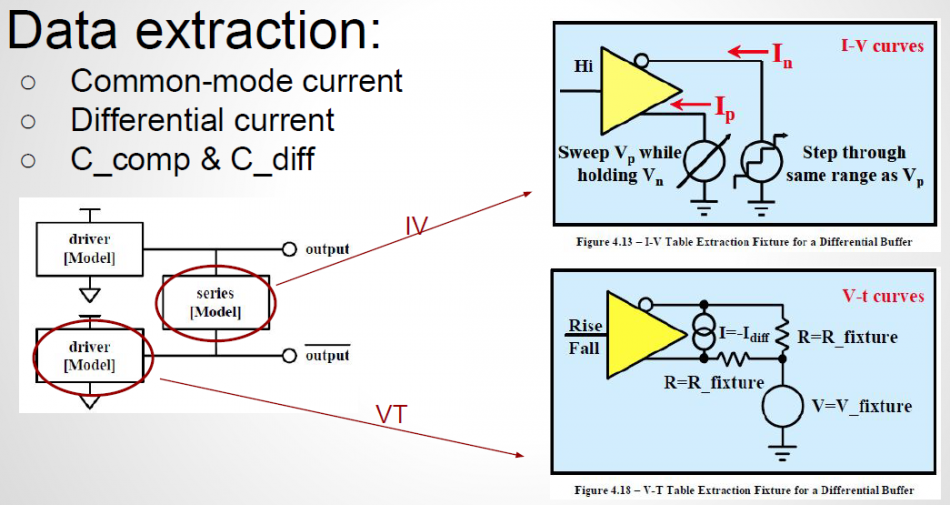• PU/PD: A two dimensional table produced by sweeping at both the P and N outputs are performed, as shown in the upper right block above. One major assumption for PU/PD extraction is that when both output pins are at the same voltage level, there will be no current flowing through the series model (i.e. the coupling portion). So in that condition, we can assume the series model does not exist at all. The current flows into or out of the buffer at that point is considered as “common-mode” current. This common-mode info is than extracted from surface table and modeled as pull-up and pull-down IV. The whole surface is then shifted vertically by subtracting this common-mode current at every x/y grids. The resulting surface table is considered as the differential current (resistance portion of the series model) and will affect the output when voltage at N and P are different. Note that to satisfy the aforementioned assumption of zero current through series element at common mode voltages, the sweep done at the P/N pins must step very slowly such that the charging/discharging of the reactive components, such as C_Comp or C_Diff can be neglected.
• PC/GC: Clamp table in IBIS are always optional. On the other hand, when a circuit have current which always exist and can’t be turned-off, these data must go to PC/GC to avoid being double counted. That is, the PC/GC table must account for current which always exist and this current will be subtracted from the PU/PD table during the modeling process to avoid being double counted. Situations like this include the ESD circuitry and/or pull-up portion of the half-differential buffer. For PC/GC extraction, common mode sweep like that for PU/PD needs to be performed while buffer is disabled (put into high-Z state). The common mode current will then be extracted as the PC/GC table. Differential mode current is disregarded as it has been accounted for in the PU/PD differential data.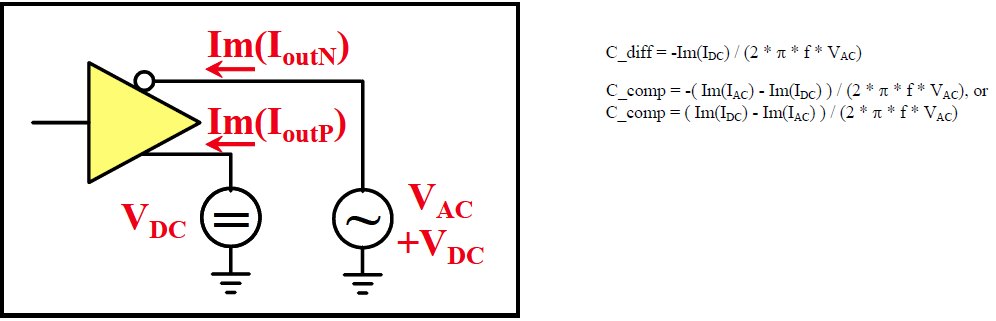• C_Comp/C_Diff: An important step here is to extract and compute the differential capacitance, C_Diff. This has to be done before VT simulation. It is because C_Diff will affect the transient state differential current, thus it must be accounted for before VT transient simulation. The extracted value will be part of the series element model by adding as C_series. In real design, the C_Comp and C_Diff are both frequency and voltage dependent. So an user can perform 2D sweep in these two dimensions and select a proper value for C_Diff. This formula to calculate c_diff/c_comp is shown above.
• VT waveform: With both static (resistive) data being processed from PU/PD/PC/GC simulation, and the reactive data, i.e. C_Diff, computed from the C_Die simulation. these two info can then be modeled together to be eliminated during VT simulation. The considerations is that: once the effect of coupling part is removed, then the remaining data can be considered as single ended and model accordingly. Having that said, reader should realize that there are much more details involved in this step. For example, how do you model these data? Two approaches may be considered:
• Use E/F/G/H control element: the surface data can be fit to minimize the mean squared error. Resulting coefficients are then realized using poly function or like of a control sources.
• Create a series element: decide the proper components and then create a series element.

Interested user may find some study presented in IBIS summit, such as [this one]

As one can see, the modeling process for a differential buffer is not linear and a certain order must be followed. Inaccurate common-mode extraction will affect IV tables and cause dc-mismatch of the steady state. In addition, failure to extract C_Diff and model different current accurately will greatly impact the accuracy of the transient data. We will see how a modeling flow should take these into account and be validated easily. These are also design concepts behind our SPIBPro flow.